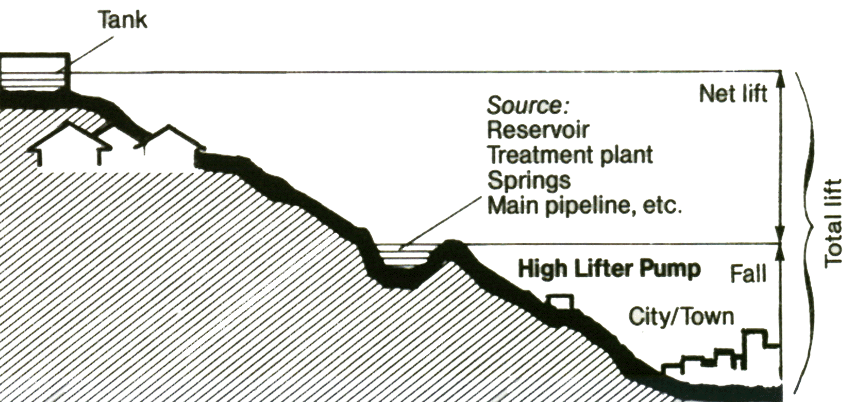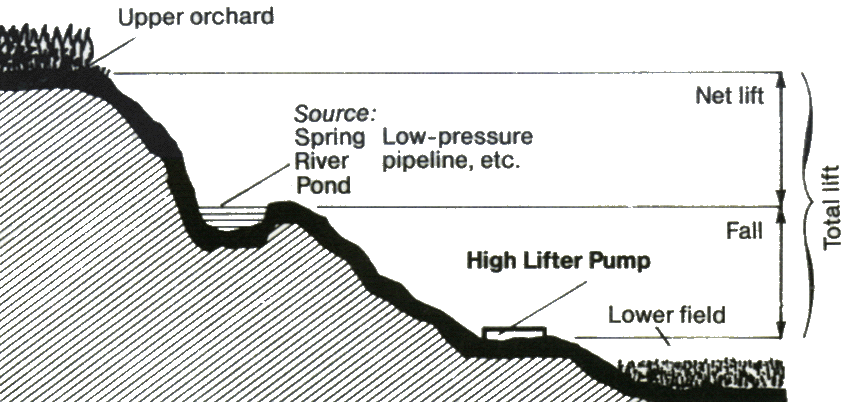How much water can I get with my 4" High Lifter water pump?Typical domestic water application using tank for reservoir and for pressure to house.High Lifter H74Typical garden/irrigation application using both pumped water and discharged water. (Tanks not necessary.)
 Do a site survey 1. Determine the height of the tank above your source (net lift)=_____________ft.2. Next determine the drop from the water source to the pump (fall)=____________ft. (Typical methods to estimate LIFT or FALL are: topographical maps, a friend with a tape measure, a hand held sight level, an altimeter, or a tube and a water pressure gauge. 1 psi = 2.3') 3. Measure or estimate the flow at the source =_________gallons per minute. (Use a gallon container and a watch.) 4. Determine the amount of water required delivery =____________gallons per day.

Performance Chart for H74To determine water delivery using chart:
 1 DETERMINE WORKING RATIO Divide Lift or Output Pressure by Fall or Inlet PressureNote: 1 psi = 2.3 foot lift or fall Inlet Pressure: 50 psi (115-foot fall) Output Pressure: 150 psi (345-foot total lift) Working Ratio = 3:1
 2 EXPRESS WORKING RATIO AS A PERCENTAGE OF PUMP RATIO. Select a pump ratio. Divide Working Ratio by Pump Ratio. Try 4:1 3 ÷ 4 = 75%
 3 SEE CHART- project a horizontal line from the Working Ratio percentage to appropriate Inlet Pressure curve. From this intersection, project a vertical line down to Inlet Flow. Inlet Flow 68 gpm
 4 CALCULATE OUTPUT FLOW: Divide Inlet Flow by Pump Ratio 68 gpm ÷ 4 = 17 gpm